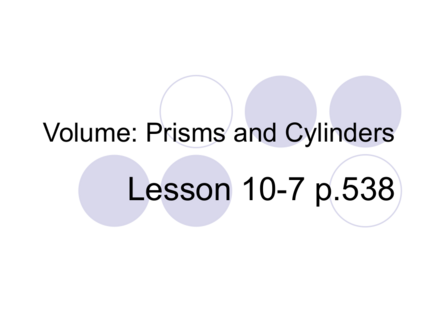# Volume of PrismContributed by:The formula for the volume of a prism is V=Bh, where B is the base area and h is the height. The base of the prism is a rectangle.
1. Volume: Prisms and Cylinders
Lesson 10-7 p.538
2. The volume of a three-dimensional figure
is the amount that fills the figure. The
volume is given in cubic units.
3. Consider this prism.
If we cut it into cubic units, it would
look like this:
2 in
3 in
4 in
4. Consider this prism.
If we cut it into cubic units, it would
look like this:
2 in
3 in
4 in
5. Consider this prism.
The common formula for finding
Volume of a rectangular prism is
V = lwh
where l is the length, w is the width
and h is the height.
2 in
3 in
4 in
6. Consider this prism.
In this example,
V = lwh = 4 (3) (2) = 24 cu. in.
2 in
3 in
4 in
7. Another way to look at this problem is take the area of the
base (meaning bottom of the figure) and multiply it by the
height. Solving in this way lets us apply the method to
other shapes as well.
Area of the base = l x w
therefore, area of the base x height
is the volume.
2 in
3 in
4 in
8.  Let’s try this method with a triangular prism
6 ft Volume = area of the base x height
The base is the triangle. A = bh
2
9 ft
A = (8) (6)
8 ft
2
A = 24 sq. ft.
Volume = area of the base x height
= 24 x 9
= 216 cu. Ft.
9. Try This
Find the volume:
4 in
5 in. 4 ft
6 in. 6 ft
3 ft
10. Try This
Find the volume:
4 in
5 in. 4 ft
6 in. 6 ft
3 ft
120 cu. in.
11. Try This
Find the volume:
4 in
5 in. 4 ft
6 in. 6 ft
3 ft
120 cu. in. 36 cu. ft.
12. This works for finding the volume of cylinders
also.
3m Volume = area of the base x height.
Area of base = r 2
5m = 3.14 x 32
= 28.26 sq. m
Volume = area of base x height.
= 28.26 x 5
= 141.3 cu. meters
13. Try This
Find the volume of the cylinder:
11 ft
5 ft.
14. Try This
Find the volume of the cylinder:
11 ft
5 ft. 1,899.7 cu. ft.
15. P.540 #1-16
16. Bellwork #
HW, red pen, book
on desk.
17. Workbook pp.86 & 87
Workbook p.89
18. Workbook p.89